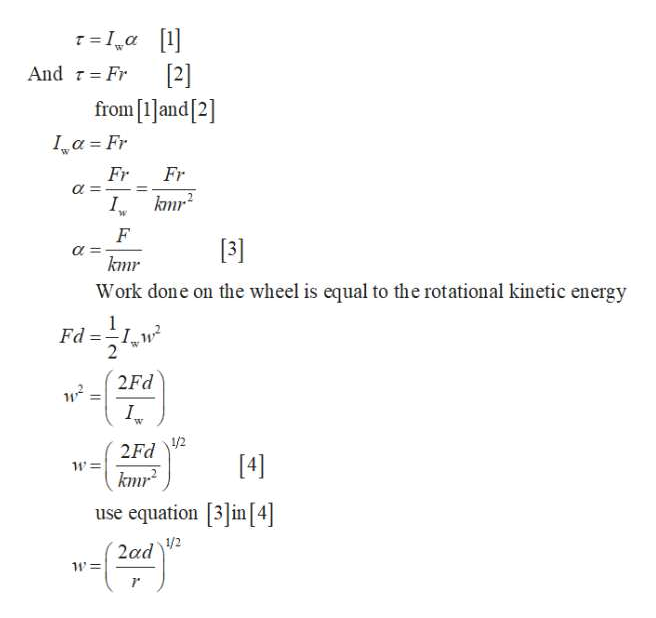# A bicycle wheel is mounted on a fixed, frictionless axle. A massless string is wound around the wheel's rim, and a constant horizontal force F of magnitude F starts pulling the string from the top of the wheel starting at time t=0 when the wheel is not rotating. Suppose that at some later time t the string has been pulled through a distance d. The wheel has moment of inertia I=kmr2, where k is a dimensionless number less than 1, m is the wheel's mass, and r is its radius. Assume that the string does not slip on the wheel.The force F  pulling the string is constant; therefore the magnitude of the angular acceleration α of the wheel is constant for this configuration.Find the magnitude of the angular velocity ω of the wheel when the string has been pulled a distance d.Express the angular velocity ω of the wheel in terms of the displacement d, the magnitude F of the applied force, and the moment of inertia of the wheel Iw.

Question
793 views

A bicycle wheel is mounted on a fixed, frictionless axle. A massless string is wound around the wheel's rim, and a constant horizontal force F of magnitude F starts pulling the string from the top of the wheel starting at time t=0 when the wheel is not rotating. Suppose that at some later time t the string has been pulled through a distance d. The wheel has moment of inertia I=kmr2, where k is a dimensionless number less than 1, m is the wheel's mass, and r is its radius. Assume that the string does not slip on the wheel.

The force F  pulling the string is constant; therefore the magnitude of the angular acceleration α of the wheel is constant for this configuration.

Find the magnitude of the angular velocity ω of the wheel when the string has been pulled a distance d.

Express the angular velocity ω of the wheel in terms of the displacement d, the magnitude F of the applied force, and the moment of inertia of the wheel Iw.
check_circle

star
star
star
star
star
2 Ratings
Step 1

Given that the magnitude of angular acceleration is constant .

Write the expression for ang...help_outlineImage TranscriptioncloseTIa [1  from and  Ia Fr And Fr Fr Fr knr I. F  kmr Work done on the wheel is equal to the rotational kinetic energy Fd I 2Fd = W 1/2 2Fd  km2 use equation in  2ad2 1'= fullscreen

### Want to see the full answer?

See Solution

#### Want to see this answer and more?

Solutions are written by subject experts who are available 24/7. Questions are typically answered within 1 hour.*

See Solution
*Response times may vary by subject and question.
Tagged in

### Physics